﻿ 基于偏最小二乘回归的环境综合治理决策分析——以日本为例

# 基于偏最小二乘回归的环境综合治理决策分析——以日本为例Decision Analysis of Environmental Comprehensive Governance Based on Partial Least Squares Regression—Take Japan as an Example

Abstract: The study of the relationship between economic development and environmental governance is of great theoretical significance and practical need. This paper studies the linear relationship between regional economic development, industrial pollution and environmental protection investment based on partial least squares regression statistical method, so as to explore the starting point and focus of regional environmental comprehensive management. The empirical analysis and model fitting of the relevant data from 2004 to 2018 in Japan show that the cumulative contribution rate of the three principal components extracted based on partial least square regression is 85.6%. In recent ten years, industrial sewage has no significant impact on the economic development of Japan, but the total investment in environmental protection and material recycling investment is significantly positively correlated with the economic development of Japan. Combined with the experience of environmental protection in Japan, it provides a theoretical basis for the formulation of regional comprehensive environmental governance policy in China.

1. 引言

Hemamala Hettige ，Ekins P.  等研究表明工业水排污在国民产出中所占的份额遵循库兹涅茨曲线轨迹。我国学者符森等  在省际经济发展数据和工业三废面板数据的研究中使用非参数估计的方法证实经济发展和环境的污染符合库兹涅茨曲线。

Euston & Liam  利用新加坡的相关数据研究表明经济发展与环保投资存在正相关的关系。而Poul Schou  通过内生性增长模型的构建认为二者既可存在负相关亦可存在正相关。叶丽娟  以我国2003~2008年的数据进行回归分析和因素分析，认为环保投资对经济增长的产出弹性与环保投资存在显著的区域差异。但总体看来，多数的相关研究结果表明环保投资对经济发展有促进作用。邵海清  采用灰色关联法计算两者间的相关度，证实环保投资对经济增长有促进作用。孙红梅等  选取2003~2013年我国30个省市的经济转型与环保投资数据构建面板向量自回归模型，得到环保投资与经济发展是相互促进的结论。

2. 多元回归方法的综合评价模型构建

2.1. 基于偏最小二乘回归的区域经济综合评价模型构建

$\mathrm{max}\left\{Cov\left({t}_{1},{u}_{1}\right)\right\}=\mathrm{max}〈{E}_{0}{w}_{1},{F}_{0}{c}_{1}〉$

$\text{s}\text{.t}\text{\hspace{0.17em}}\left\{\begin{array}{l}{w}_{1}^{\text{T}}{w}_{1}=1\\ {c}_{1}^{\text{T}}{c}_{1}=1\end{array}$

${E}_{0}^{\text{T}}{F}_{0}{F}_{0}^{\text{T}}{E}_{0}{w}_{1}={\theta }_{1}^{2}{w}_{1}$

${E}_{0}^{\text{T}}{F}_{0}{F}_{0}^{\text{T}}{E}_{0}{w}_{1}={\theta }_{1}^{2}{w}_{1}$

${E}_{0}={t}_{1}{p}_{1}^{\text{T}}+{E}_{1}$

${F}_{0}={t}_{1}{r}_{1}^{\text{T}}+{F}_{1}$

${p}_{1}=\frac{{E}_{0}^{\text{T}}{t}_{1}}{{‖{t}_{1}‖}^{2}}$${r}_{1}=\frac{{F}_{0}^{\text{T}}{t}_{1}}{{‖{t}_{1}‖}^{2}}$

${t}_{2}={E}_{1}{w}_{2}$${u}_{2}={F}_{1}{c}_{2}$

${E}_{1}={t}_{2}{p}_{2}^{\text{T}}+{E}_{2}$${F}_{1}={t}_{2}{r}_{2}^{\text{T}}+{F}_{2}$

${p}_{2}=\frac{{E}_{1}^{\text{T}}{t}_{2}}{{‖{t}_{2}‖}^{2}}$${r}_{2}=\frac{{F}_{1}^{\text{T}}{t}_{2}}{{‖{t}_{2}‖}^{2}}$

${F}_{0}={t}_{1}{r}_{1}^{\text{T}}+\cdots +{t}_{A}{r}_{A}^{\text{T}}+{F}_{A}={E}_{0}\left[\underset{j=1}{\overset{A}{\sum }}{w}_{j}^{*}{r}_{j}^{\text{T}}\right]+{F}_{A}$

3. 经济发展、环保投资以及工业污染之关系评估——以日本为例

3.1. 数据来源及处理

3.2. 日本经济与环保产业概况统计分析

1970年至1980年，日本直接用于治理环境的财政预算经费增长了1.3倍。到了80年代，日本开始多渠道整治污染，对环境极为重视。如今日本工业的单位GDP能耗与我国相比不足我国的14%。经过多年不懈地环境治理，日本无论是在节能减排还是循环经济等领域上，都取得了巨大的成就。下表(表1)为日本1971~2015年，日本年度国民生产总值、环保投资总额和工业废渣排放量的数据统计表。

Table 1. The Japan’s data of GDP, total investment in environmental protection and industrial waste 1971-2015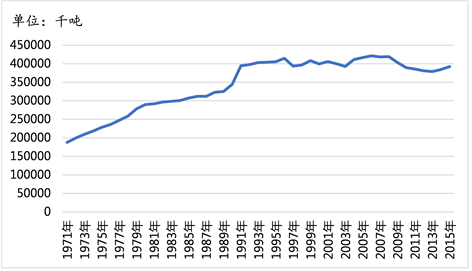Figure 1. Japan’s industrial waste discharge statistics data 1971-2015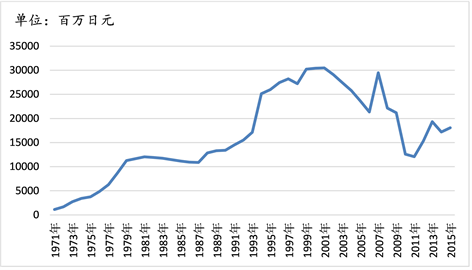Figure 2. Japan’s total environmental protection investment statistics data 1971-2015

3.3. 经济发展与环境治理关系评估指标选取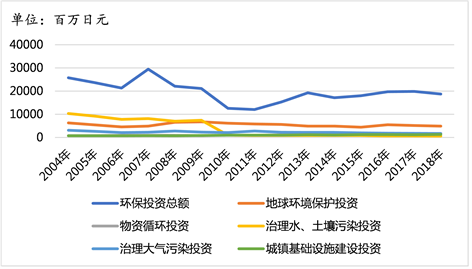Figure 3. Japan’s environmental protection investment statistics data 2004-2018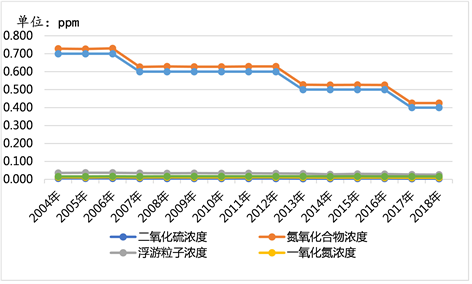Figure 4. Japan’s statistics on atmospheric pollution data 2004-2018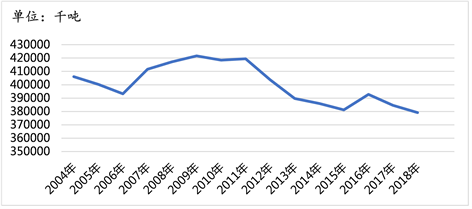Figure 5. Japan’s industrial waste discharge statistics data 2004-2018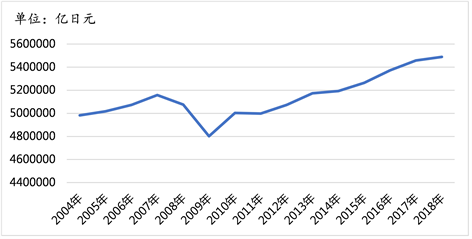Figure 6. Japan’s GDP statistics data 2004-2018

3.4. 数据预处理

Table 2. Model of Japan’s economic development, industrial sewage and environmental protection investment regression 2004-2018

Table 3. Japan’s economic development, industrial sewage and environmental protection investment explanatory variables normalization data 2004-2018

4. 基于偏最小二乘回归的日本经济发展环保治理关系实证分析Table 4. Japan related data of partial least squares regression model principal component analysis table

${Y}_{2}=0.312{t}_{1}+0.204{t}_{2}+0.387{t}_{3}$

$\begin{array}{c}{Y}_{2}=0.142{w}_{1}+1.865{w}_{2}+0.262{w}_{3}+0.09{w}_{4}-0.253{w}_{5}-0.239{w}_{6}-0.334{q}_{1}\\ \text{\hspace{0.17em}}\text{\hspace{0.17em}}+1.179{q}_{2}+0.377{q}_{3}-0.339{q}_{4}-0.392{q}_{5}+0.004{q}_{6}-0.174{q}_{7}\end{array}$ (4-1)Table 5. Japan’s related data of partial least squares regression model variance analysis tableTable 6. Japan’s related data of partial least squares regression model residual statistics table

5. 结果分析及政策建议

5.1. 结果分析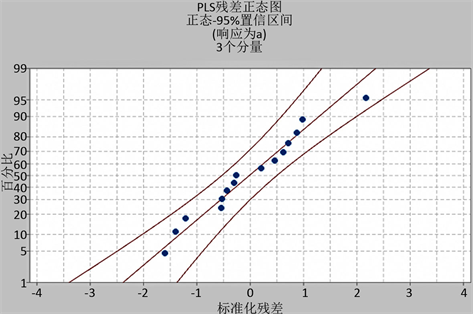Figure 7. Standardized residual normality map of Japan’s related data partial least squares regression model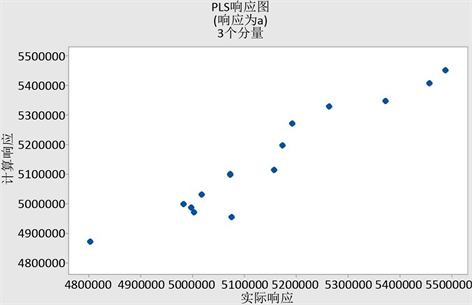Figure 8. Japan’s related data of partial least squares regression model PLS response map

5.2. 政策建议

 Hettige, H., Mani, M. and Wheeler, D. (2000) Industrial Pollution in Economic Development: The Environmental Kuznets Curve Revisited. Journal of Development Economics, 62, 445-476.
https://doi.org/10.1016/S0304-3878(00)00092-4

 Ekins, P. (1997) The Kuznets Curve for the Environment and Economic Growth: Examining the Evidence. Environment and Planning A, 29, 805-829.
https://doi.org/10.1068/a290805

 符淼, 黄灼明. 我国经济发展阶段和环境污染的库兹涅茨关系[J]. 中国工业经济, 2008(6): 35-43.

 Quah, E. and Boon, T.L. (2003) The Economic Cost of Particulate Air Pollution on Health in Singapore. Journal of Asian Economics, 14, 73-90.
https://doi.org/10.1016/S1049-0078(02)00240-3

 Schou, P. (2000) Polluting Non-Renewable Resources and Growth. Environmental and Resource Economics, 16, 211-227.
https://doi.org/10.1023/A:1008359225189

 叶丽娟. 环保投资对区域经济增长影响的差异研究[D]: [硕士学位论文]. 广州: 暨南大学, 2011.

 邵海清. 环保投资与国民经济增长的灰色关联分析[J]. 生产力研究, 2010(12): 14-15.

 孙红梅, 赵正丽. 经济转型与环保投资相关性的实证研究——基于面板向量自回归模型和空间面板模型的实证分析[J]. 山西财经大学学报, 2016, 38(12): 16-28.

 周涛. 我国环保投资结构对工业污染治理绩效影响研究[D]: [硕士学位论文]. 杭州: 浙江理工大学, 2014.

 张平淡, 朱松, 朱艳春. 环保投资对中国SO2减排的影响——基于LMDI的分解结果[J]. 经济理论与经济管理, 2012(7): 84-94.

 游士兵, 严研. 逐步回归分析法及其应用[J]. 统计与决策, 2017(14): 31-35.

 王慧文. 偏最小二乘回归方法及其应用[M]. 北京: 国防工业出版社, 1999

 El Jabri, M., Sanchez, M.-P. and Trossat, P. (2019) Comparison of Bayesian and Partial Least Squares Regression Methods for Mid-Infrared Prediction of Cheese-Making Properties in Montbéliarde Cows. Journal of Dairy Science, 102, 6943-6958.
https://doi.org/10.3168/jds.2019-16320

 姚天宝, 申惟文, 任建民. 逐步回归和偏最小二乘回归模型在混凝土重力坝变形监测中的应用[J]. 水电能源科学, 2015, 33(2): 81-84.

 肖雪梦, 张应应. 三种回归方法在消除多重共线性及预测结果的比较[J]. 统计与决策, 2015(24): 75-78.

 王鲁明. 区域循环经济发展模式研究[D]: [硕士学位论文]. 青岛: 中国海洋大学, 2005.

 裴瑱. 国外环保产业政策及其借鉴[J]. 政策瞭望, 2015(8): 51-52.

 王欢. 河北省环保投资对工业废气排放影响的实证研究[D]: [硕士学位论文]. 石家庄: 河北经贸大学, 2017.

 王玲. 环境经济政策下环保投资的中日对比研究[D]: [硕士学位论文]. 北京: 中央民族大学, 2012.

 胡世前. 中国经济增长与工业污染互动关系研究[J]. 财经问题研究, 2015(6): 19-25.

Top